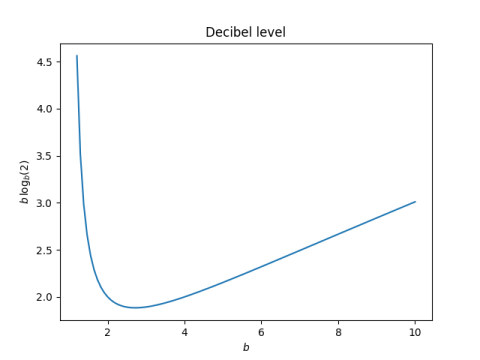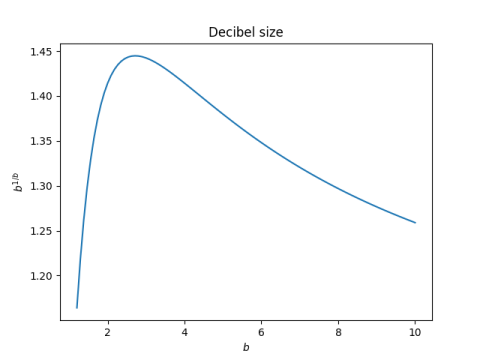# The base with the largest decibel

This post is an expansion on a comment by Nathan Hannon:

My favorite definition of e is “the base whose version of the decibel is the largest”.

I hadn’t seen that before. Sounds interesting. What does it mean?

## First attempt

My first thought was that the statement meant that the function

b logb(x)

is largest when b = e.

It’s easier to do calculus with natural logs—that’s one reason advanced math almost exclusively uses natural logs—and so we’ll rewrite the expression above as

b log(x) / log(b)

where log without a subscript is natural log. If we differentiate this expression with respect to b, we get

(log(b) – 1) log(x) / (log(b))²

and this expression is zero, independent of x, when log(b) = 1, i.e. when b = e.

So to recap, for any fixed x, the expression

b logb(x)

as a function of has a critical point where b = e.

So that’s the maximum, right? No, it’s a minimum!The value of x doesn’t change the shape of the curve and I arbitrarily chose x = 2 in the plot.

## Second attempt

Looking back at the original statement, it says e is the base that maximizes the decibel, not the level measured in decibels. The unit in which levels are minimized is the one which maximizes the size of the unit. The original statement is right and my first reading of it was backward.

As I wrote about here, the function

b logb(x)

is equivalent to the logarithm base b1/b. This base is one “decibel,” using quotes because we’ve replaced the 10’s in the definition of decibel with an arbitrary base b. The base, the decibel unit, is maximized when b = e.## One thought on “The base with the largest decibel”

1. MikeD

Is that another way of showing the same phenomenon that numbers in base e are the most efficient (in information theoretic terms) way to express the value. I think it’s something like “radix efficiency” or something like that where you measure how many digits it takes to represent a number versus the entropy in each digit.